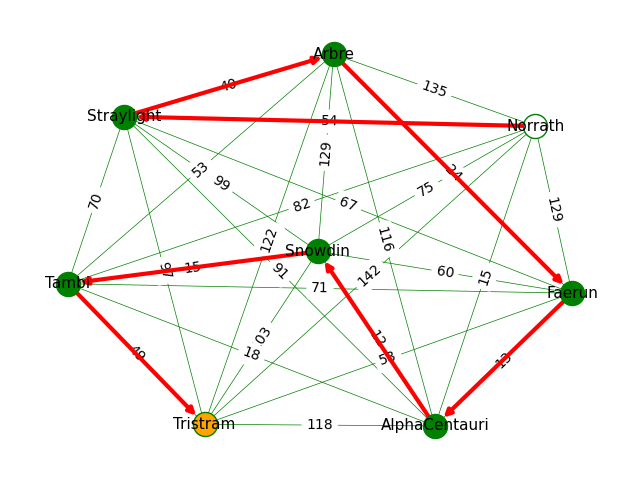# Learning Python with Advent of Code Walkthroughs

Dazbo's Advent of Code solutions, written in Python

# The Python Journey - Creating and Visualising Graphs with NetworkX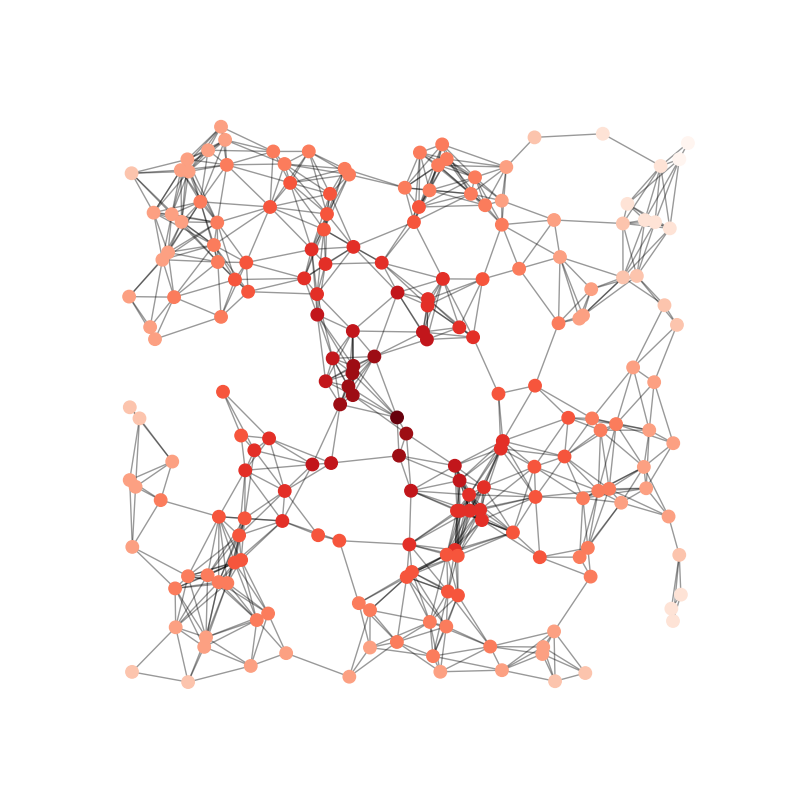## Introduction

NetworkX is a cool library that allows us to create, manipulate, interrogate, and render graphs. It can take a lot of the pain out of building graphs. It also has cool methods that allow us to do things like: find the shortest path from a to b. And it can also render the graph as an image for us.

## Installing NetworkX

``````py -m pip install networks
``````

## NetworkX Guides and Tutorials

Here are a few useful links:

## Getting Started With NetworkX

We need to import the relevant packages. You’ll probably want to import `matplotlib.pyplot` if you want to render any graph visualisations.

``````import networkx as nx
import matplotlib.pyplot as plt
``````

## Supplying Graph Data

Typically, we’ll want to build a graph by supplying edge information. I.e. a connection between one node and another.

For example, let’s say we have a list of input data, where each line of data contains two locations. We might build an unweighted, undirected graph like this:

``````def build_graph(data) -> nx.Graph:
""" Build graph from list of connected points.

Args:
data (list[str]): pairs of points, in the form "Name_X connected to Name_Y"

Returns:
dict: (start, end) = distance
"""
graph = nx.Graph()
points_match = re.compile(r"^(\w+) connected to (\w+)")

# Add each edge, in the form of a location pair
for edge in data:
start, end = points_match.findall(edge)

return graph
``````

Or, if we also have some sort of weight information (e.g. distance between points, cost of a route, etc), then we can build a directed graph like this:

``````def build_graph(data) -> nx.Graph:
""" Build graph from list of connected points.

Args:
data (list[str]): pairs of points, in the form "Name_X to Name_Y = distance_n"

Returns:
dict: (start, end) = distance
"""
graph = nx.Graph()
distance_match = re.compile(r"^(\w+) to (\w+) = (\d+)")

# Add each edge, in the form of a location pair
for edge in data:
start, end, distance = distance_match.findall(edge)
distance = int(distance)

return graph
``````

Or we could be a directed weighted graph, like this:

``````def build_graph(rules):
""" Builds a graph from a dict of rules

Args:
rules (dict): Look like... 'shiny plum': {'dotted beige': 5, 'faded orange': 1}

Returns:
nx.DiGraph: Directed graph
"""
graph = nx.DiGraph()
for parent, children in rules.items():
for child, child_count in children.items():
return graph
``````

We can also create our edges from various data structures, such as from a `Pandas` `DataFrame`, using `nx.from_pandas_edgelist()`.

## Drawing the Graph

Having built a graph, we can then render it as a visualisation.

The basic functon is `draw()`.

E.g.

``````nx.draw(graph, with_labels=True, node_color='blue')
``````

Note that NetworkX must decide where to position each node on the drawing canvas. This is done by supplying a layout to NetworkX. If you don’t supply one, NetworkX uses ‘spiral’ by default.

There are two ways you can supply a layout.

1. You can supply a layout and obtain on the positions. You then use these positions in later calls.
2. You can use the `draw_xxx()` method, where `xxx` is one of the supported layouts.

In the following examples, I’ll render directed, weighted output with the following data, and using a number of different layouts.

``````light red bags contain 1 bright white bag, 2 muted yellow bags.
dark orange bags contain 3 bright white bags, 4 muted yellow bags.
bright white bags contain 1 shiny gold bag.
muted yellow bags contain 2 shiny gold bags, 9 faded blue bags.
shiny gold bags contain 1 dark olive bag, 2 vibrant plum bags.
dark olive bags contain 3 faded blue bags, 4 dotted black bags.
vibrant plum bags contain 5 faded blue bags, 6 dotted black bags.
faded blue bags contain no other bags.
dotted black bags contain no other bags.
``````

### Spring Layout (Default)

``````def draw_graph(graph):
nx.draw(graph, edge_color="grey", width=1, with_labels=True)
ax = plt.gca()
ax.set_axis_off()
plt.show()
``````

Or equivalent:

``````def draw_graph(graph, searching_for, ancestors):
pos = nx.spring_layout(graph)
nx.draw(graph, pos=pos, edge_color="grey", width=1, with_labels=True)
ax = plt.gca()
ax.set_axis_off()
plt.show()
``````

Output: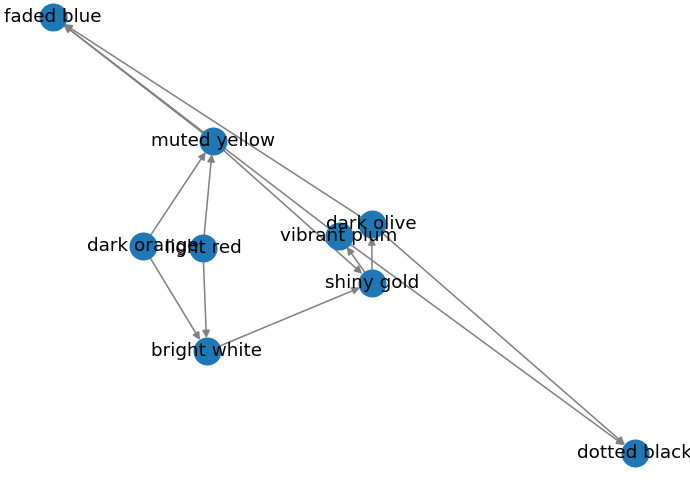### Spiral Layout

``````pos = nx.spiral_layout(graph)
nx.draw(graph, pos=pos, edge_color="grey", width=1, with_labels=True)
``````

Output: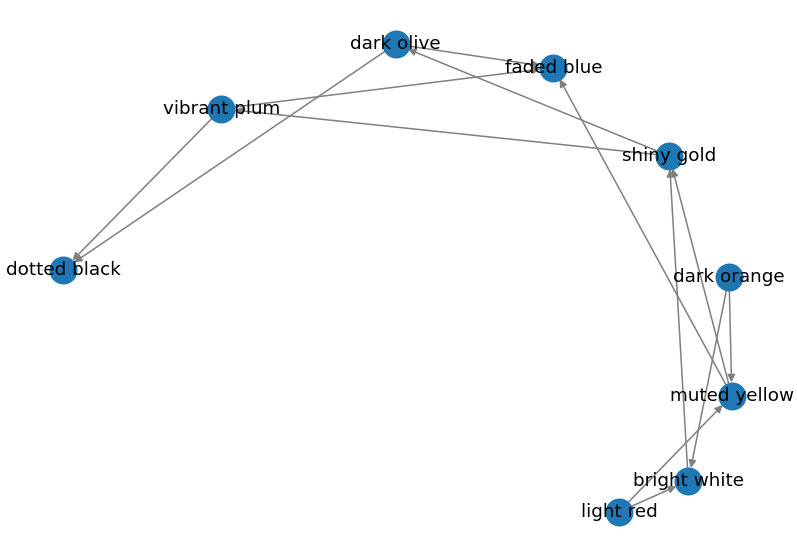### Circular Layout

``````pos = nx.spiral_layout(graph)
nx.draw(graph, pos=pos, edge_color="grey", width=1, with_labels=True)
``````

Output: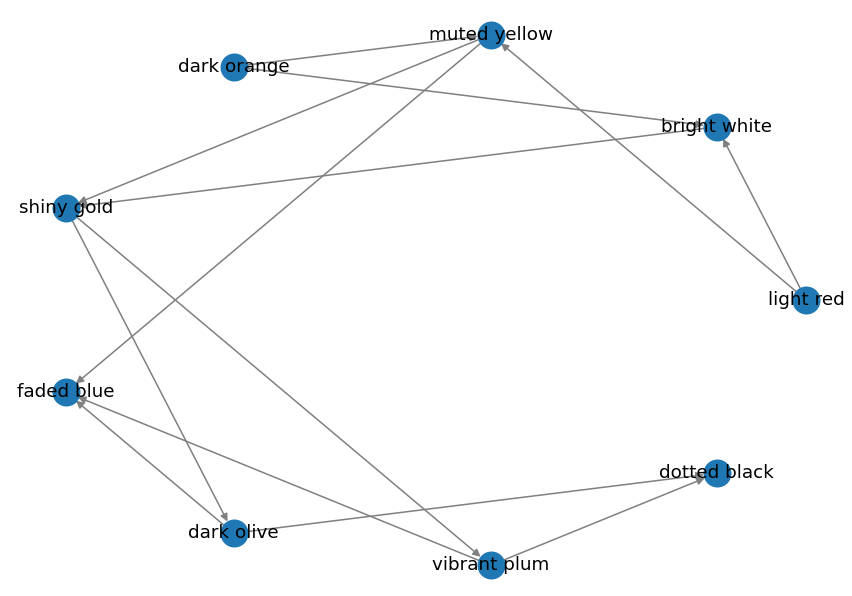### Planar Layout

``````pos = nx.spiral_layout(graph)
nx.draw(graph, pos=pos, edge_color="grey", width=1, with_labels=True)
``````

Output: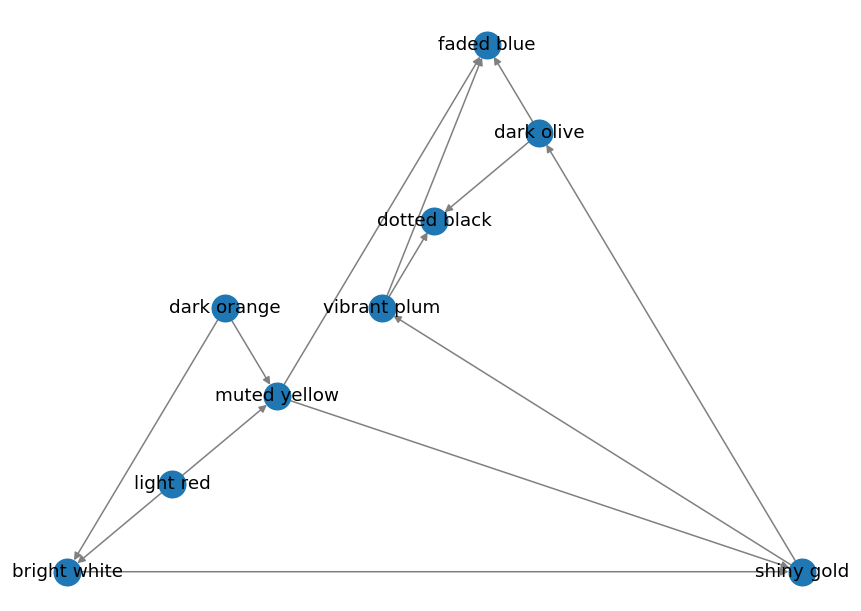### Random Layout

``````pos = nx.spiral_layout(graph)
nx.draw(graph, pos=pos, edge_color="grey", width=1, with_labels=True)
``````

Output: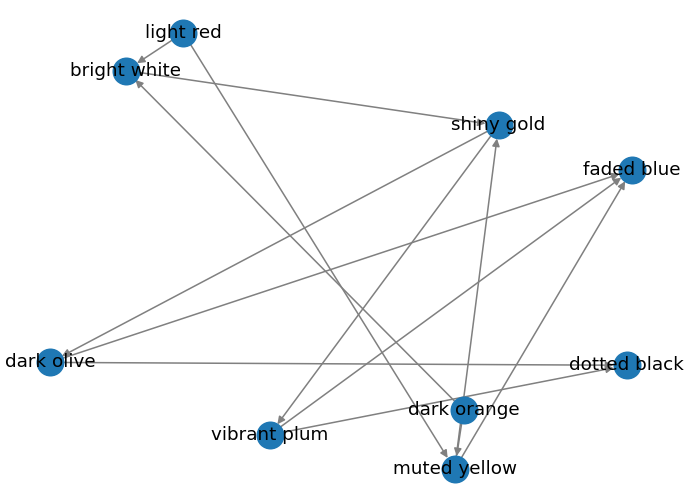## More Interesting Graphs

The graphs above are quite boring. So let’s look at how we can make them more interesting and valuable.

### Colouring Nodes Based on Attributes

Here we examine each node in the supplied graph, and then colour the nodes based on specific attributes.

• The the node is one of START or END, we colour it in green.
• If the node is one of a list of nodes called `special_nodes`, then we colour it blue.
• Otherwise, we colour it red.

This works by creating a list of `colours`, which has the same number of elements as `graph.nodes`, and is in the same order as the nodes. We must pass this list to our `draw()` method.

``````def render(graph, START, END, special_nodes, file):
_ = plt.subplot(121)  # throwaway variable
pos = nx.spring_layout(self._graph)

# set colours for each node in the array, in the same order as the nodes
colours = []
for node in graph.nodes:
if node in (START, END):
colours.append("green")
elif node in special_nodes:
colours.append("blue")
else:
colours.append("red")

nx.draw(graph, pos=pos, node_color=colours, with_labels=True)

dir_path = Path(file).parent
if not Path.exists(dir_path): # Create output folder if it doesn't exist
Path.mkdir(dir_path)
plt.savefig(file)   # save the visualisation as a file
``````

The output looks like this: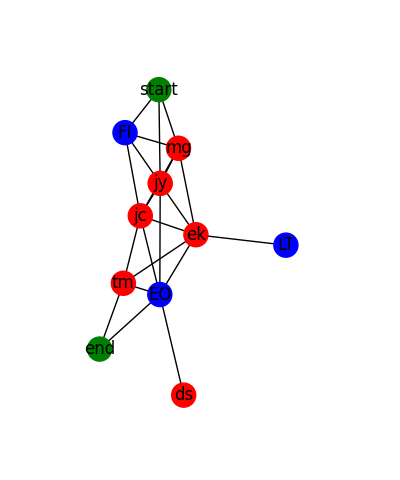### Colouring a Particular Path

In the example below, we:

• Draw a weighted graph that shows a number of locations as nodes, and a number of edges as distances between nodes.
• We also superimpose a specific route that we want to highlight.

To achieve this, we:

• Draw all the nodes in green, except the start and end nodes of our route. (Note that the locations in the `route` must be nodes from the graph itself.)
• Label all the nodes.
• Add the start and end nodes from our route, colouring them white and orange, respectively.
• Draw all the edges, with a thin green line.
• Add the edge labels, i.e. the distances of each edge. (Remember that this is a weighted graph, and the weight of each edge is the distance.)
• Finally, we redraw all the edges from our route, with direction, with heavy red lines. We use the convenience method `nx.utils.pairwise(route)` to obtain the edges between each adjacent node in the supplied `route`.
``````def draw_graph(graph, route):
start_node = route
end_node = route[-1]

pos = nx.spring_layout(graph) # create a layout for our graph

# Draw all nodes in the graph
nx.draw_networkx_nodes(graph, pos,
nodelist=route[1:-1], # exclude start and end
node_color="green")

# Draw all the node labels
nx.draw_networkx_labels(graph, pos, font_size=11)

# Draw start and end nodes
nx.draw_networkx_nodes(graph, pos, nodelist=[start_node],
node_color="white", edgecolors="green")
nx.draw_networkx_nodes(graph, pos, nodelist=[end_node],
node_color="orange", edgecolors="green")

# Draw closest edges for each node only - with thin lines
nx.draw_networkx_edges(graph, pos,
edge_color="green", width=0.5)

# Draw all the edge labels - i.e. the distances
nx.draw_networkx_edge_labels(graph, pos, nx.get_edge_attributes(graph, DISTANCE))

# Draw the edges that make up this particular route
route_edges = list(nx.utils.pairwise(route))
nx.draw_networkx_edges(graph, pos, edgelist=route_edges,
edge_color="red", width=3, arrows=True)

ax = plt.gca()
plt.axis("off")
plt.tight_layout()
plt.show()
``````

The visualisation looks like this: